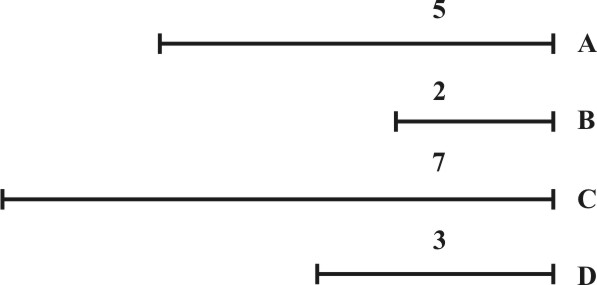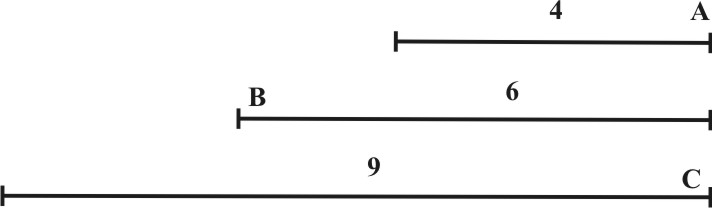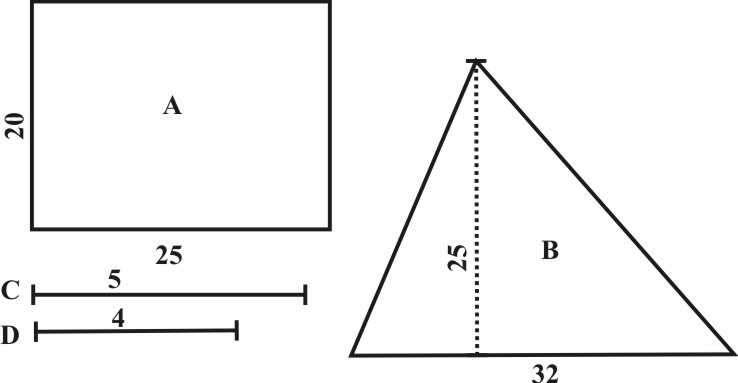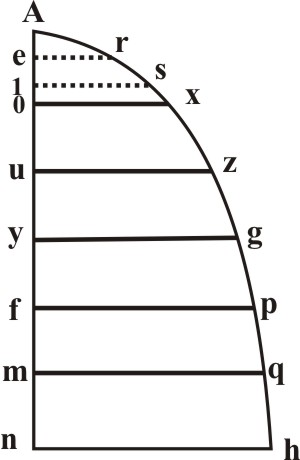The First Book of the Sector

1. To find a Proportion between two or more Superficies.

Take one of the sides of the greater Superficies given, according to it open the Sector in the points of 100 and 100 in the Lines of Superficies, then take the like sides of the lesser Superficies severally, and carry them parallel to the former, till they stay in like points, so the number of points wherein they stay, shall shew their Proportion unto 100.Let A and B, be the sides of like Superficies, as the sides of two Sqaues, or the Diameters of two Circles, first I take the side A, and to it open the Sector in the points of 100; then keeping the Sector to this Angle, I enter the lesser side B, parallel to the former and find it to cross the Lines of Superficies in the points of 40, wherefore the Proprtion of the Superficies, whose side is A, to that whose side is B, is as 100 unto 40, which is in lesser number as 5 unto 2.

This Proportion might have been wrought by 60, or any other Number that admits several Division. It may also be wrought without opening the Sector, for if the sides of the Suoerficies given applied to the Lines of Superficies, beginning always at the Center of the Sector, there will be such Proportion found beteew them, as between the number of parts whereon they fall.

3. To diminish a Superficies in a given Proportion.

Take the side of the Superficies, and to it open the Sector in the points of the numbers given; then keeping the Sector at that Angle, the parallel distance between the points of the number required, shall give the side of the Superficies required.

Let A be the side of a Square, to be augmented in the Proportion of 2 to 5. First, I take the side A, and put it over in the Line of Superficies in 2 and 2; so the parallel between 5 and 5, doth give me the side B, on which if I should make a Square, it would have such Proportion to the Square of A, as 5 unto 2.

In like manner, if B were the Semidiameter of a Circle to be dimished in the Proportion of 5 unto 2, I would take out B, and put it over the Lines of Superficies in 5 and 5; so the parallel between 2 and 2 would give me A; on which Semidiameter if I should make a Circle, it would be less than the Circle made upon the Semidiameter B, in such Proportion as 2 is less than 5.

For variety of work, the like caution may be here observed to that which we gave in the third Proposition of Lines.

5. To substract one like Superficies from another.

First, the Proportion between like sides of the Superficies given, is to be found by the first Proposition of Superficies, then add or subtract the numbers of those Proportions, and accordingly augment or diminish by the former Proposition.As if A and B were the side of two Squares, and it wre required to make a third Square equal to them both. First the proposition between the Squares of A and B, would be found to be as 100 unto 40, or in the lesser numbers as 5 to 2; then because 5 and 2 added do make 7, I augment the side A in the Proportion of 5 to 7, and it will produce the side C, on which if I make a Square, it will be equal to both Squares of A nad B, which was required.

In like manner A and B being the sides of two Squares, if it were required to substract the Square f B, out of the Square of A, and to make a Square equal to the Remainder, here the Proportion being as 5 to 2, because 2 taken out of 5, the Remainder is 3, I would diminish the side A in the Proportion of 5 to 3, and so I should produce the side D, on which if I make a Square, it will be equal to the Remainder, when the Square of B is taken out od the Square of A, that is, the two Squares made upon B and D, shall be equal to the first Square made upon the side A.

6. To find a mean Proportion between two Lines given.

First find what Proportion is between the Lines given, as they are Lines, by the fifth Proposition of Lines, then open the Sectroe in the Lines of Superficies, according to his Number, to the quantity of the one, and a parallel taken between the points of the Number belonging to the other Line shall be the mean Proportional.Let the Lines given be A and C. The Proportion between them (as they are Lines) will be found, by the fifth Proposition of Lines, to be, as 4 unto 9. Wherefore, I take the Line C, and put it over to the Lines of Superficies between 9 and 9, and keeping the Sector at this Angle, his Parallel between 4 and 4 doth give me B, and put over between 9 and 9: so his Parallel between 4 and 4, shall give me the first Line A. Whereby it is plain, that these three Lines do hold in continual Proportion; and therefore B is a mean Proportional between A and C, the extremes given.

Upon the finding out of this mean Propotion, depend many Corellaries, as

To make a Square equal to a Superficies given.

It the Superficies given be a rectangle Parallelogram, a mean proportional between the two unequal Sides shall be the Side of his equal Square.

If it shall be a triangle, a mean Proportion between the perpendicular and half the Base shall be the Side of his equal Square. If it shall be any other right-lined Figure, it may be resolved into triangles, and so a Side of a Square found equal to every Triangle, and these being reduced into one equal Square, it shall be equal to the whole right-lined Figure given.

To find a Proportion between Superficies, though they be unlike one to the other.

If to every Superficies we find the side of his equal Square, the proportion between these Squares shall be the Proportion between the Superficies given.Let the Superficies be the oblong A, and the Triangle B. First between the unequal Sides of A, I find a mean proportional, and note it in C: This is the side of a Square equal unto A. Then between the Perpendicular of B and half his Base, I find a mean Proportional, and note it in D: this is the side of a Square equal to B: but the propoertion between the Squares of C and D, will be found, by the first Proposition of Superficies to be as 5 unto 4: and therefore this is the Proportion between those given Superficies.

To make a Superficies, like to one Superficies, and equal to another.

Let the one Superficies given be the Triangle A, and the other the Rhomboides B; and let it be required to make another Rhomboides like to B, and equal to the Triangle A.

First, between the Perpendicular and the Base of B, I find a mean Proportional, and note it in B, as the side of his equal Square, then between the Perpendicular of the Triangle A, and half his Base, I find a mean proportional, and note it in A, as the side of his equal Square. Wherefore now as the side B is to the siedA, so shall the sides of the Rhomboides given be to C and D, the sides of the Rhomboides required, and his Perpendiculaer also to E, the Perpendicular required.Having the Sides and the Perpendicular, I may frame the Rhomboides up, and it will be equal to the Triangle A.

If the Superficies given had been any other right-lined Figure, they might have been resolved into triangles and then brought into Squares as before.

Many such Corollaries might have been annexed, but the means of finding a mean Proportional being known, they all follow of themselves.

7. To find a mean proportional between two Numbers given.

First, reckon the two Numbers given on both sides of the Lines of Superficies, from the Center, and mark the terms whereunto they extend; then take a Line out of the Line of Lines, or any other Scale of equal parts resembling one of those Numbers given, and put it over in the terms of his like Number in the Line of Superficies; for so keeping the Sectror at this Angle, the Parallel taken from the terms of the other Number and measuredin the same Scale from which the other parallel wa taken, shall shew the Mean Proportional which was required.

Let the Numbers given be 4 and 9. If I shall take the Line A in the Diagram of the sixth Proposition resembling 4, in the Scale of equal parts, and to it open the Sectror in the terms of 4 and 4, in the Line of Superficies, his Parallel between 9 and 9 doth give me B for the Mean Proportional. And this measured in the Scale of equal parts doth extend to 6, wich is the Mean Proportional Numer between 4 an 9. For as 4 to 6, so 6 to 9.

In like manner, if I take the Line C, resembling 9, in a Scale of equal parts, and to it open the Sector in the terms of 9 and 9, in the Line of Superficies, his Parallel between 4 and 4 doth give me the same Line B, which will prove to be 6, as before, if it be measured in the same Scale whence C was taken.

For the Figures 1, 2, 3, 4 &c, here set down upon the Line, do sometime signifie themselves alone: sometime 12, 20, 30, 40, &c some time 100, 200, 300, 400, &c. and so forward, as the matter shall require. The first Figure of every Number is always that which is here set down: the rest must be supplied according to the nature of the Question.

If you suppose Pricks under the Number given (as in Arithmetical Extraction) and the last Prick to the left hand shall fall under the last Figure (which will be as oft as there be odd Figures) the unite will be best placed at 1, in the middle of the Line; so the Root and the Square will both fall forward, toward the end of the Line. But, if the last Prick shall fall under the last Figure but one (which will be as oft as there be even Figures) then the unite may be placed at 1 in the beginning of the Line, and the Squrae in the second length: or the unite may be placed at 10, in the end of the Line, so the Root and the Squrae will both fall bachward, toward the middle of the Line.

9. The Root being given, to find the Square Number of that Root.

In : the Extraction of a Square Root it is usual to set Pricks under the first Figure, the thrid, the fifth, the seventh, and so forward, beginning from the right hand to ward the left, and as many Pricks as fall to be under the Square Number given, so many Figures shalt be in the :Root: so that if the Number given be less than 100, the root shall be only one Figure; if less than 10000, it shal be but two Figures; if less than 100000, it shall be three Figures, &c.

There upon the Lines of Superficies are divided first into an hundred parts, and if the Number given be greater than 100, the first Division (which before did signify only one) must signify 100, and the whole Line shall be 10000 parts: if yet the number given be greater than 10000, the first Division must now signify 10000; and the whole Line be esteemed at 1000000 parts: and if this be too little to express the Number given, as oft as we have to recourse to the beginning, the whole Line shall increase itself an hundered times.

By these means if the last Prick to the left hand shall fall under the last Figure, which will be as oft as there be odd Fifures, the Number given shall fall out between the Center of the Sector and the thenth Division: but if the last Prick shall fall under the last Figure but one, which will be as oft as there are even Figures, then the Number given shall fall out between the tenth Division and the end of the Sector.

This being considered, when a Number is given, and the Square Root is required, take a pair of Compasses, and setting one Foot in the Center, extend the other to term the number given in one of the Lines of Superficies; for this Distance applied to one of the Lines of Lines, shall shew what the Square Root is, without opening the Sector.

Thus 36 give a Root of 6; and 360, a Root of almost 19: and 3600 a Root of 60; and 36000, a Root os 189, &c.

In like manner, the nearest Root of 725 is found to be (about) 27, the nearest Root of 7250, is about 85: the nearest of 72500, about 269: the nearest Root of 725000, about 851: And so in the rest.

On the contrary, a Number given may be squared, if first we extend the Compasses to the Number given in the Line of Lines, and then apply that Distance to the Lines of superficies, as may appear by the fomer example.

10. Three Numbers given, to find the fourth in a duplicated Proportion.

It is plain (by Euclid. Lib. 6, Prop. 19 & 20.) that like Superficies doth hold in a duplicated Proportion of their homologal Sides, whereupon a question being moved concerning Superficies and their Sides: It is usual in Arithmetick, that the Proportion be first duplicated before the Question be resolved, which is not necessary in the Use of the Sector, only the Numbers which do signify Superficies, must be reckoned in the Lines of Superficies, and they which signifie the Sides of Superficies, in the Line of Lines, after this manner.

If a Question be made concerning a Superficies, the two Numbers of the first Denomination must be reckoned in the Line of Lines: and the Sector opened in the terms of the first Number to the quantity of a Line out of the Scale of Superficies resembling the second Number; so his Parallels taken between the terms of the third Number, being measured in the same Scale of Superficies, shall give the Superficial Number which was required.

As if a Square, whose Side is 40 Perches in length, shall contain 10 Acres in the Superficies, and it be required to know how many Acres the Square should contain, whose side is 60 Perches. Here if I took 10 out the Line of Superficies, and put it over 40, in the Line of Lines, his Parallel between 60 and 60, measured in the Line of Superficies, would be 22 ½, and such is the number of Acres required. For Squares do hold in a duplicated Proportion of their sides; wherefore when the Proportion of their sides is 4 to 6, and 4 multiplied into 4 become 16, and 6 multiplied into 6 become 36, the Proportion of their Squares shall be as 16 to 36, and such is the Proportion of 10 to 22 ½.

If a Field measured whith a Statute Perch of 16 ½ foot, shall contain 288 Acres, and it be required to know how many Acres it would contain if it were measured with a Woodland perch of 18 foot.

Here because the Proportional is reciprocal, if I took 288 out of the Line of Superficies, and put it over in 18 in the Line of Lines, his Paralllel between 16 ½ and 16 ½ measured in the Line of Superficies, would be 242; and such is the Number of Acres requiered.

For seeing the Proportion of the Sides is as 16 ½ to 18, or in lesser Numbers as 11 to 12, and that 11 multiplied into 11 become 121, and 12 into 12 become 144, the Proportion of these Superficies shall be as 121 to 144, and so have 288 to 242, in reciprocal Proportion.

On the contrary, if a question be proposed concerning the Side of a Superficies, the two Numbers of the first Denomination must be reckoned in the Line of Superficies, and the Sector opened in the terms of the first Number to the quantity of a Line, out of the Line of Lines or some Scale of equal parts, resembling the second Number; so his Parallel taken between the terms of the third Number being measured in the same Scale with the second Number, shall give the fourth Number required.

As if a Field containeth 288 Acres when it was measured with a Statute Perch of 16 ½, and being measured with another Perch, was found to contain 242 Acres, it were required to know what was the length of the Perch with which it was measured.

Here because the proportion is reciprocal, if I took 16 ½ out of the Line of Lines, and put it over 242 in the Line of Superficies, his Parallel between 288 and 288, being measured in the Line of Lines, would be 18, and such ist the length of the perch (in Feet) wherewhich the Filed was last measured.

For seeing the proportion of the Acres is as 288 unto 242, or in lesse Numbers, as 144 to 121, and that the Root of 144 is 12, and the Root of 121 is 11, the Proportion of Roots, and consequently of the perches, shall be as 12 to 11, and so are 16 ½ to 18 in reciprocal Proportion.

If 360 men were to be set in form of a long Square, whose Sides shall have the Proportion of 5 to 8; and it were required to know the Number of men to be placed in front and file: If the Sides were only 5 and 8, there should be but 40 men; but there are 360: therefore working as before, I find that,

• As 40 to the Square of 5: So 360 to the Square of 15.
• As 40 to the Square of 8: So 360 to the Square of 24.

And so 15 and 24 are the Sides required.

If 1000 men were lodged in a square ground whose Side were 60 paces, and it were required to know the Side of the Square wherein 5000 might be lodged, here working as before, I should find that,

• As 1000, are to the Square of 60: So 5000 to the Square of 134.

And such, very near, is the Number of paces required.

11. How to describe a Parabola, by help of the Line of Lines and Superficies.Upon A n as the Diameter, prick down, by the Line of Lines, the equal Parts A0, Au, Ay, Af, Am, An, &c. and from these Points raise the Perpendicular 0 x, uz, yg, fp, mq, nh, &c. And upon the Perpendicular 0x, assume the Point x, and open the Sector in the Line of Superficies, so that 0x (being the first Perpendicular) may fall in with the Points 1...1 (the first of the Line of Superficies: Then if you take off from the same Line 2...2, you shall prick down uz, and 3...3 gives yg; and 4...4, fp; 5...5, mq; 6...6, nh; &c.

Or, you may begin your work from nh, which (because it is the sixth Perpendicular) take from n to h, the Point assumed, and set that length in the Line of Superficies from 6 to 6, so may you prick down the other Points correspondingly.

Through these Points h, q, p, g, with an even hand draw the parabola.

And here note, that Parabola's may be described of infinite Variety, according to the Cones from whence the are taken, yet keeping all one and the same length.© Rainer Stumpe URL: www.rainerstumpe.de